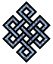fail2ban bad ip database: ip 82.112.32.45

| ip database | live view | stats | report | help | api key:

 ip: 82.112.32.45 hostname: 82-112-32-45.k-telecom.org country:[RU] Russian Federation first reported: 24.09.2018 14:08.05 GMT+0200 last reported: 05.02.2019 16:44.11 GMT+0200 time period: 134d 03h 36m 06s total reports: 11 reported by: 3 host(s) filter(s): ssh (8) ssh (3) tor exit node no badips.com db Lookupport scan of '82.112.32.45':

[-hide]
```# Nmap 6.40 scan initiated Mon Sep 24 14:09:02 2018 as: /usr/bin/nmap -sU -sS -O 82.112.32.45
Nmap scan report for 82-112-32-45.k-telecom.org (82.112.32.45)
Host is up (0.073s latency).
Not shown: 1000 open|filtered ports, 995 filtered ports
PORT     STATE  SERVICE
22/tcp   open   ssh
80/tcp   open   http
443/tcp  closed https
1723/tcp open   pptp
3306/tcp closed mysql
No exact OS matches for host (If you know what OS is running on it, see http://nmap.org/submit/ ).
TCP/IP fingerprint:
OS:SCAN(V=6.40%E=4%D=9/24%OT=22%CT=443%CU=%PV=N%G=Y%TM=5BA8D44E%P=x86_64-pc
OS:-linux-gnu)SEQ(SP=F9%GCD=1%ISR=10A%TI=Z%CI=I%TS=8)OPS(O1=M5B4ST11NW7%O2=
OS:M5B4ST11NW7%O3=M5B4NNT11NW7%O4=M5B4ST11NW7%O5=M5B4ST11NW7%O6=M5B4ST11)WI
OS:N(W1=7120%W2=7120%W3=7120%W4=7120%W5=7120%W6=7120)ECN(R=Y%DF=Y%TG=40%W=7
OS:210%O=M5B4NNSNW7%CC=Y%Q=)T1(R=Y%DF=Y%TG=40%S=O%A=S+%F=AS%RD=0%Q=)T2(R=N)
OS:T3(R=N)T4(R=Y%DF=Y%TG=40%W=0%S=A%A=Z%F=R%O=%RD=0%Q=)T5(R=Y%DF=Y%TG=40%W=
OS:0%S=Z%A=S+%F=AR%O=%RD=0%Q=)T6(R=Y%DF=Y%TG=40%W=0%S=A%A=Z%F=R%O=%RD=0%Q=)
OS:T7(R=N)U1(R=N)IE(R=Y%DFI=N%TG=40%CD=S)

OS detection performed. Please report any incorrect results at http://nmap.org/submit/ .
# Nmap done at Mon Sep 24 14:10:54 2018 -- 1 IP address (1 host up) scanned in 112.54 seconds
```
```Σ = 56 | Δt = 0.004021167755127s
```